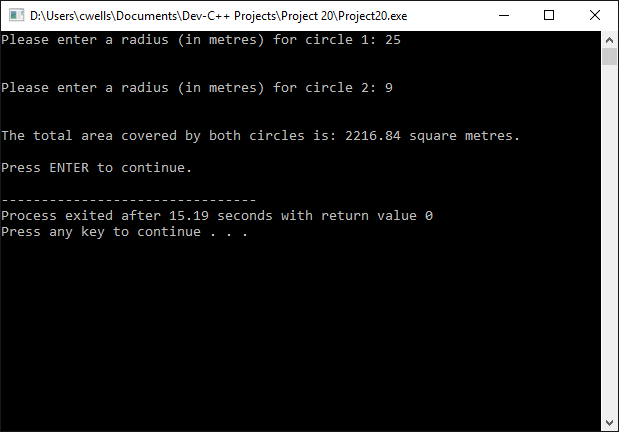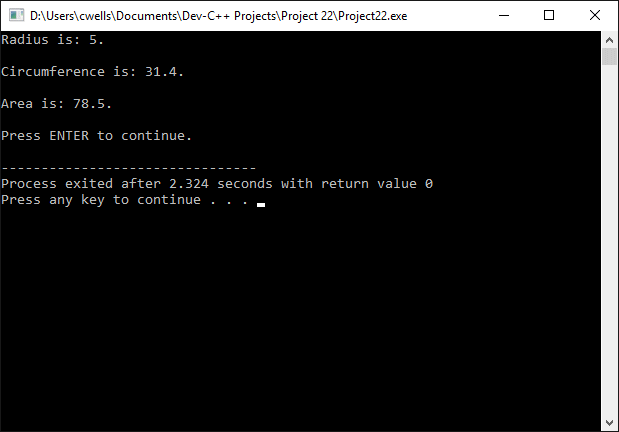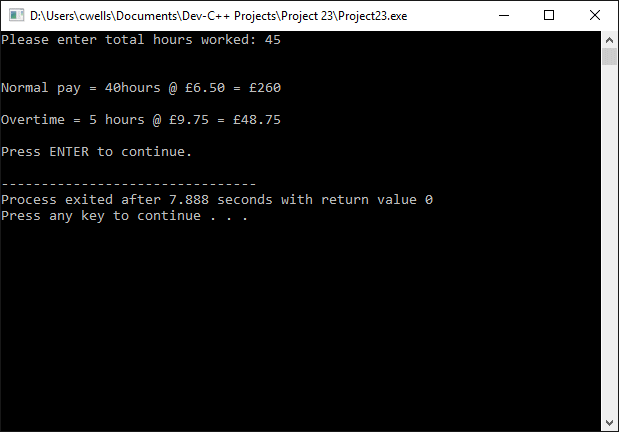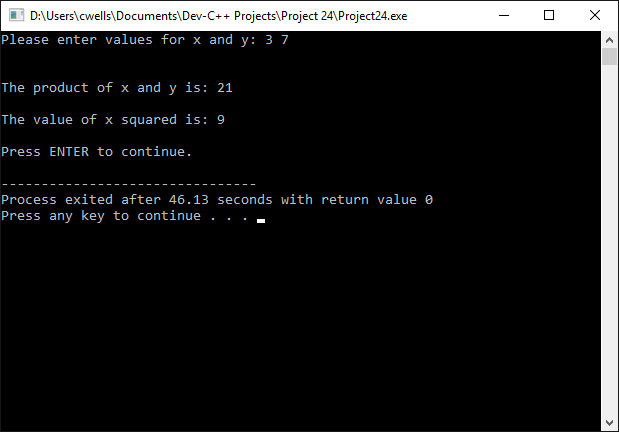Functions

A function consists of a block of program code that can be called from any point in a program. A function can accept parameters (or arguments), and can return a value to routine that calls it. The general format of a function is shown below.

type name (parameter1, parameter2, ...) {statements}

The type component specifies what data type (if any) will be returned to the calling routine by the function, while the name of the function must be used when the function is called. Each parameter consists of a data type specifier followed by a parameter name, which will be used by the function's code to reference the parameter, in the same way it would reference a local variable. If there are two or more parameters, they are separated by commas. The function's statements form the body of the function, and are enclosed within curly braces. The short program below demonstrates how a function may be created and used.

// Example Program 1

#include <iostream>
using namespace std;

/* the function "circ" accepts a floating point value
as a parameter, and returns a floating point value */

{
return 2 * rad * 3.14;
}

int main ()
{
string s;
float circumference;

cout << "circumference is: " << circumference << ".";
cout << "\n\nPress ENTER to continue.";
getline( cin, s );
return 0;
}The output from example program 1

Note that, although we have declared and defined the circ() function ahead of the main() function in the code, program execution always starts with main(). When the function is called by main(), program execution moves to the circ() function, which executes the code within the function body. Once the function has completed its assigned task, which is to calculate the circumference of a circle based on the radius of the circle (which is passed to it as a parameter), it returns the calculated value to the calling procedure (main()). Program execution then continues form the program statement that follows the function call in main().

Note that variables declared within a function body have local scope only. In other words, they are not visible outside the function in which they are declared. In the example program above, therefore, it would not be possible for the circ() function to refer directly to the variable radius, since it is local to the function main(). We can, however, pass a copy of the variable radius to the function as a parameter. If we want variables to be accessible from anywhere in the program, we must declare them as global variables, i.e. outside of any function. Global variables normally appear before any function declarations in the code.

A function call can be used within an expression in the same way as a variable, in the sense that it's return value can be used directly. The following short program illustrates the use of a function in this way.

// Example Program 2

#include <iostream>
#include <sstream>
using namespace std;

/* This program calculates and adds together the area of two circles for which the radius is provided by the user. The "area" function accepts a floating point value as a parameter, and returns a floating point value */

{
}

int main ()
{
string s;
cout << "Please enter a radius (in metres) for circle 1: ";
getline( cin, s );
cout << "\n\nPlease enter a radius (in metres) for circle 2: ";
getline( cin, s );
cout << "\n\nThe total area covered by both circles is: ";
cout << total_area << " square metres.";
cout << "\n\nPress ENTER to continue.";
getline( cin, s );
return 0;
}The output from example program 2

In the above program, we have made two calls to the area() function in the same statement, the return values of which are added together and assigned to the variable total_area.

Functions with no return value

It is sometimes the case that we need to create a function that performs some task, but does not return a value. Because the function declaration requires that we specify a type, the void keyword is used to specify that there is no return value. The general format of such a function is shown below.

void name (parameter1, parameter2, ...) {statements}

It is equally possible that we could declare a function that takes no parameters. We must still provide the parentheses following the name of the function, but in this case they will be empty. The format of a function that returns no value, and accepts no parameters, is shown below.

void name () {statements}

In some programming languages, this type of function might be called a procedure rather than a function, since these languages draw a distinction between a program routine that returns a value to its calling process, and one that doesn't. The following short example program uses a function that simply displays a message on the screen.

// Example Program 3

#include <iostream>
using namespace std;

void display_message()
{
cout << "Hello World!";
}

int main ()
{
string s;

display_message();
cout << "\n\nPress ENTER to continue.";
getline( cin, s );
return 0;
}The output from example program 3

Passing variables by reference

In the functions we have created thus far, any variables passed to those functions as arguments have been passed by value. In other words, a copy of the variable has been passed to the function. Whatever actions are carried out by the function cannot, therefore, change the value of the variable itself. There may be occasions, however, when we want the function to change the value of one or more external variables.

One method would be to assign the function's return value to the variable. This would work if only one variable needs to be changed, since a function can only directly return one value. Another way would be to declare the affected variables as global, so that the function could access them directly. Unfortunately, this method would make them accessible by other parts of the program, and is not considered good programming practice. The short program below demonstrates how variables may be passed to a function by reference.

// Example Program 4

#include <iostream>
using namespace std;

void circle(float& radius, float& circumference, float& area)
{
circumference = 2 * radius * 3.14;
}

int main ()
{
string s;

cout << "Circumference is: " << circumference << ".\n\n";
cout << "Area is: " << area << ".";
cout << "\n\nPress ENTER to continue.";
getline( cin, s );
return 0;
}The output from example program 4

Note that, in the declaration of the function circle(), the type of each parameter was followed by an ampersand (&). This tells us that, rather than passing a copy of the variable to the function (i.e. passing by value), what we are passing to the function here is the address of the variable. In other words, we are providing the function with a pointer to the variable, so that it can manipulate the variable itself. Any changes made to the value of these variables within the function remain in effect after the function has returned control of program execution to the calling process., and any further reference to these variables by the program's code will reflect this. One of the main advantages of passing variables by reference in this manner is that it allows us to create functions that can, effectively, return multiple values.

Using default values in function parameters

A function declaration can include default values for one or more parameters, which are used if the corresponding parameter is left blank in the function call. The assignment operator is used to set a default value in the parameter list. If a different value is specified by the function call, it will be used instead of the default value. The short program below demonstrates the use of default values.

// Example Program 5

#include <iostream>
using namespace std;

float wage_calc(float hours, float hourly_rate = 6.5)
{
return hours * hourly_rate;
}

int main ()
{
string s;
float normal_hours, overtime, total_hours;

cout << "Please enter total hours worked: ";
cin >> total_hours;
getline( cin, s );
if (total_hours > 40)
{
normal_hours = 40;
overtime = total_hours - 40;
}
else
{
normal_hours = total_hours;
overtime = 0;
}
cout << "\n\nNormal pay = " << normal_hours << "hours @ \x9c";
cout << "6.50 = \x9c" << wage_calc(normal_hours);
cout << "\n\nOvertime = " << overtime << " hours @ \x9c" << "9.75";
cout << " = \x9c" << wage_calc(overtime, 9.75);
cout << "\n\nPress ENTER to continue.";
getline( cin, s );
return 0;
}The output from example program 5

In the above program there are two calls to the function wage_calc(). In the first call, the second parameter is left blank, so the default value of 6.5 is used to calculate the return value. In the second call, a value of 9.75 is supplied as a parameter, and this is used to calculate the return value instead of the default parameter value.

In C++, two functions may share the same name so long as their parameter lists differ in terms of either the type of the parameters passed, or the number of parameters. The process of declaring functions with the same name, but that exhibit different behaviours depending on the arguments passed to them, is known as overloading. The short program below demonstrates this facility.

// Example Program 6

#include <iostream>
using namespace std;

int multiply(int x, int y)
{
return x * y;
}

int multiply(int x)
{
return x * x;
}

int main ()
{
string s;
int x, y;

cout << "Please enter values for x and y: ";
cin >> x >> y;
getline( cin, s );
cout << "\n\nThe product of x and y is: " << multiply(x, y);
cout << "\n\nThe value of x squared is: " << multiply(x);
cout << "\n\nPress ENTER to continue.";
getline( cin, s );
return 0;
}The output from example program 6

In the above program, functions have the same name (multiply()), but one version accepts two integer arguments, while the other accepts only one. The compiler determines which one to use by looking at the number of parameters used in the function call. The function that takes two integer arguments returns the product of the arguments, while the function that takes only one argument returns the square of the argument.

Inline functions

The inline specifier tells the compiler to insert the function's code directly into the program at the point where the function call occurs, rather than handling it as a procedure call. This action does not change the behaviour of the function. It simply insert's the function's code at every point in the code where the function call occurs. This has the disadvantage of increasing the overall size of the program, but allows the program to execute faster, since the overhead involved in making a procedure call has been eliminated. The general format for the declaration of an inline function is shown below.

inline type name ( parameter list ) { statements }

Note that, if the inline keyword has been used in the function declaration, it does not need to be used when making subsequent calls to the function. Note also that many compilers already generate inline code if this will result in optimal program performance. The inline specifier simply indicates to the compiler the programmer's preference for the function to be treated as inline code.

Recursive functions

Recursive functions are functions that call themselves, and are often used to carry out repetitive tasks such as sorting lists or calculating the factorial of a number. In order to calculate the factorial of the number six (6!), for example, we could use the following expression:

6! = 6 * 5 * 4 * 3 * 2 * 1 = 720

The short program below uses a recursive function to calculate the factorial of a number entered by the user.

// Example Program 7

#include <iostream>
using namespace std;

long factorial (long number)
{
if (number > 1)
{
return number * factorial (number - 1);
}
else
{
return 1;
}
}

int main ()
{
string s;
long x;

cout << "Please enter a number: ";
cin >> x;
getline( cin, s );
cout << "\n\nThe factorial of x is: " << factorial (x);
cout << "\n\nPress ENTER to continue.";
getline( cin, s );
return 0;
}The output from example program 7

The recursive function factorial() calls itself repeatedly until the exit condition (that the number passed to the function as a parameter is not greater than 1) is met. Since each successive call to factorial() passes the input parameter of the previous call minus one as an argument, the exit condition is certain to be satisfied at some point. It is essential, when using recursive functions, to ensure that there is an exit condition that will be satisfied within a finite number of iterations, since failure to do so will result in an infinite loop that will eventually result in a stack overflow.

Function prototypes

So far, in all of the example programs in this section, we have defined functions before they are called from within the main() function. This is because a function cannot be used by a program until it has been declared. We can, however defer the writing of the function body until later in the program, providing we provide a function prototype. The prototype simply determines function's name, together with its return type and parameter types. The general form of a function prototype is shown below.

type name (parameter_type1, parameter_type2, ...);

The main difference between this and the examples we have seen hitherto is the complete absence of the function body (i.e. the program statements, enclosed in curly braces, that determine what the function actually does). Note that the inclusion of parameter names is optional for the function prototype; only the parameter types need to be declared. The example program below uses two functions, which are declared as function prototypes ahead of the main() function, and defined later in the code.

// Example Program 8

#include <iostream>
#include <sstream>
using namespace std;

// function prototypes
float calc_area(float);
float calc_circ(float);

int main ()
{
string s;

cout << "Please enter a radius (in metres) for the circle: ";
getline( cin, s );
cout << "\n\nThe area of the circle is: ";
cout << area << " square metres.";
cout << "\n\nThe circumference of the circle is: ";
cout << circ << " metres.";
cout << "\n\nPress ENTER to continue.";
getline( cin, s );
return 0;
}

// function definitions